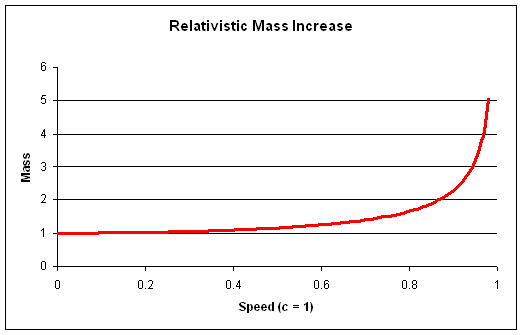# Red shift once more

Hi,
so last time I was writing about Dopplers effect and I was using normal Dopplers effect but actually I forgot something very important and those are relativistic speeds.

Relativistic speed is the speed when Newtonian physics is no longer able to accurately predict what is going to happen. This can happen from 1 to 10% of speed of light. This border is hard to define since it is hard to define when Newtonian physics is not enough accurate.On the left you can see graph of increasing mass when you increase speed.

That is one of many relativistic effect, this also means that when you are walking you are heavier. Also another effect is time dilation for example.

Time dilation is the problem with red shift in Universe. You can not just use equations like I used before but you need to use special relativity with time dilation, since the thing is speeding away from you and the time runs differently for you and it.This equation is the right one, more accurate for huge speeds with which you will be in astronomy probably dealing with.

Lambdas are the wave lengths of the one you are observing and the real one of the object. V is velocity and c speed of light, z is the red shift.

So I checked with one problem which I found on the internet. It was about quasar far away which is moving in high speeds. The red shift is 0.158.

So i used this calculator to find out what is v/c which was 0.14656. With this I found out what is the speed which was 43,695 km/s. Then I put it in the equation above and the red shift was about 0.15777 (something). So I can see that I did it right and this equation is truly giving us the red shift.

Dragallur

1st picture
2nd picture is from czech wiki article about relativistic Doppler effect